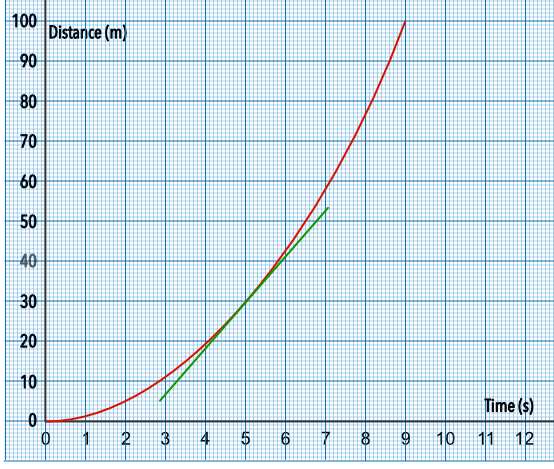Velocity

## Velocity

On a distance-time graph, the gradient of the graph gives the velocity of the object being measured:

velocity = frac(text(distance))(text(time))## Example 1

From the distance-time graph, above, what is the velocity of the car at 5 seconds? Give your answer correct to 1 decimal place.

The velocity is the gradient = frac(text(change in distance))(text(change in time))

Take a tangent at 5 seconds

= frac(52.5 - 37)(7 - 3)

= 3.875 ms-1

## Example 2

Using the graph, what is the average velocity for the first 100m? Give your answer correct to 1 decimal place.

Distance covered in 100m took 9 seconds

= frac(100)(9)

= 11.11 ms-1